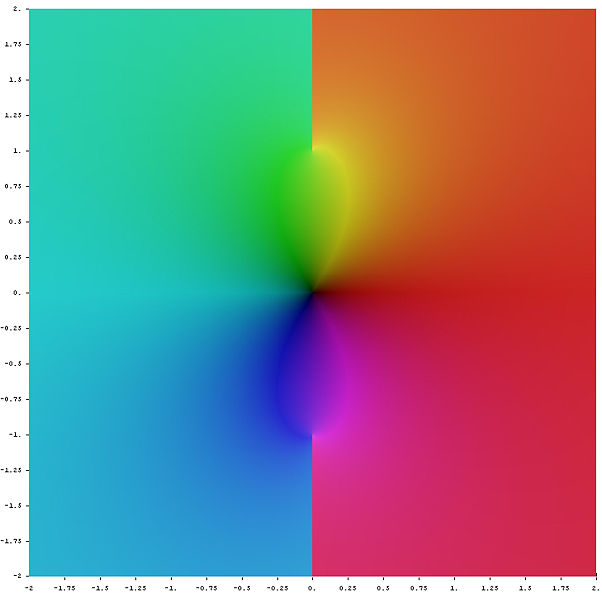# ArcTan Dilemma

Consider the Maclaurin's series expansion of $tan^{-1}(x)$.

$tan^{-1}(x)=x-\frac{x^{3}}{3}+\frac{x^{5}}{5}...................\infty$

If you didn't know how we got here:Maclaurin Series

Differentiating with respect to $x$ on both sides we get,

$\frac{1}{1+x^{2}} = 1-x^{2}+x^{4}-.........................\infty$

The right hand side is clearly an infinite geometric series with a common ratio between consecutive terms as $-x^{2}$. By the knowledge of geometric series we can say that the right hand side should simplify to give the left hand side. If you didn't know that prove it to yourself after seeing the following wiki:

Geometric Progressions

Note that the result for the sum of an infinite geometric series is under the condition that the absolute value of the common ratio is less than 1, which would imply that the series converges. But if you notice something here you can see that this expression for the left hand side holds true for all $x$ in the domain of $tan^{-1}(x)$ but the right hand side doesn't. There is a limiting condition for the right hand side. Hence I seem to want to believe that this is some sort of Deja Vu and I can't seem to account for this. Could anyone please help me in this venture of mine to understand the intricacies of math? If you do, please drop a comment below. Your help will be genuinely appreciated. Cheers!

By the way if you ever wondered what the $tan^{-1}(z)$ would be, where $z$ is a complex number, here you go........ I thought it would make the note colourful. Does it?.Note by Anirudh Chandramouli
5 years, 1 month ago

This discussion board is a place to discuss our Daily Challenges and the math and science related to those challenges. Explanations are more than just a solution — they should explain the steps and thinking strategies that you used to obtain the solution. Comments should further the discussion of math and science.

When posting on Brilliant:

• Use the emojis to react to an explanation, whether you're congratulating a job well done , or just really confused .
• Ask specific questions about the challenge or the steps in somebody's explanation. Well-posed questions can add a lot to the discussion, but posting "I don't understand!" doesn't help anyone.
• Try to contribute something new to the discussion, whether it is an extension, generalization or other idea related to the challenge.

MarkdownAppears as
*italics* or _italics_ italics
**bold** or __bold__ bold
- bulleted- list
• bulleted
• list
1. numbered2. list
1. numbered
2. list
Note: you must add a full line of space before and after lists for them to show up correctly
paragraph 1paragraph 2

paragraph 1

paragraph 2

[example link](https://brilliant.org)example link
> This is a quote
This is a quote
    # I indented these lines
# 4 spaces, and now they show
# up as a code block.

print "hello world"
# I indented these lines
# 4 spaces, and now they show
# up as a code block.

print "hello world"
MathAppears as
Remember to wrap math in $$ ... $$ or $ ... $ to ensure proper formatting.
2 \times 3 $2 \times 3$
2^{34} $2^{34}$
a_{i-1} $a_{i-1}$
\frac{2}{3} $\frac{2}{3}$
\sqrt{2} $\sqrt{2}$
\sum_{i=1}^3 $\sum_{i=1}^3$
\sin \theta $\sin \theta$
\boxed{123} $\boxed{123}$

Sort by:

The given series expansion of $\tan^{-1} x$ holds true for $|x|<1$. This is why the equation that you got by differentiating both sides is valid only for $|x|<1$. Hope this helps.

- 5 years, 1 month ago

why does the series hold true for $|x|<1$?

- 5 years, 1 month ago

Use the ratio test.

- 5 years, 1 month ago

Actually I realised now that the Cauchy convergence test itself is enough. I say itself mainly because I feel that it is easy

- 5 years, 1 month ago

Did you mean this test?

- 5 years, 1 month ago

yep

- 5 years, 1 month ago

by the way if wikipedia failed to make you understand the cauchy convergence test, read that book about hyperreals i gave you chapter 9

- 5 years, 1 month ago

Ok. Will do. But personally, I feel more at home with standard real analysis. :)

- 5 years, 1 month ago

by the way could you send the proof using ratio test. I am intrigued as I don't seem to be getting the limit of consecutive terms

- 5 years, 1 month ago

For the ratio test, the limit of the modulus of the consecutive terms is required, which is 1. Hence, radius of convergence is 1.

- 5 years, 1 month ago

oh yes i didn't take the mod value I took the value of consecutive terms as it is thanks

- 5 years, 1 month ago

Consider the $nth$ term of the series expansion of $\tan^{-1} x$. It is given by $(-1)^{n-1} \frac{x^{2n-1}}{2n-1}$

If the series is to convege, then the $nth$ term should convege to zero. If $|x|>1$, then the $nth$ term diverges as $n \to \infty$. If $|x|<1$, then the $nth$ term tends to zero. Hence, a necessary condition for thhe series to convege is that $|x|<1$. It can be shown that this condition is also sufficient for convergence of the series.

- 5 years, 1 month ago

Dude u may know this famous series 1 -1/3 +1/5 -1/7 ...... Which sums up to $\pi$ / 4.

And if we substitute 1 in the tan inverse expansion we get the same series.

- 5 years, 1 month ago

Yes. But that requires more justification.

- 5 years, 1 month ago

Say r is some real number. Then LaTeX:$\frac{r}{\sqrt{r^{2}+1}}$ lies between -1 and 1. So you can plug in that value into the series.

- 3 years, 7 months ago